# 2.6: 求解线性不等式

•• OpenStax
• OpenStax
$$\newcommand{\vecs}{\overset { \rightharpoonup} {\mathbf{#1}} }$$ $$\newcommand{\vecd}{\overset{-\!-\!\rightharpoonup}{\vphantom{a}\smash {#1}}}$$$$\newcommand{\id}{\mathrm{id}}$$ $$\newcommand{\Span}{\mathrm{span}}$$ $$\newcommand{\kernel}{\mathrm{null}\,}$$ $$\newcommand{\range}{\mathrm{range}\,}$$ $$\newcommand{\RealPart}{\mathrm{Re}}$$ $$\newcommand{\ImaginaryPart}{\mathrm{Im}}$$ $$\newcommand{\Argument}{\mathrm{Arg}}$$ $$\newcommand{\norm}{\| #1 \|}$$ $$\newcommand{\inner}{\langle #1, #2 \rangle}$$ $$\newcommand{\Span}{\mathrm{span}}$$ $$\newcommand{\id}{\mathrm{id}}$$ $$\newcommand{\Span}{\mathrm{span}}$$ $$\newcommand{\kernel}{\mathrm{null}\,}$$ $$\newcommand{\range}{\mathrm{range}\,}$$ $$\newcommand{\RealPart}{\mathrm{Re}}$$ $$\newcommand{\ImaginaryPart}{\mathrm{Im}}$$ $$\newcommand{\Argument}{\mathrm{Arg}}$$ $$\newcommand{\norm}{\| #1 \|}$$ $$\newcommand{\inner}{\langle #1, #2 \rangle}$$ $$\newcommand{\Span}{\mathrm{span}}$$$$\newcommand{\AA}{\unicode[.8,0]{x212B}}$$

##### 学习目标

• 在数字线上绘制不等式图
• 求解线性不等式
• 将单词翻译成不等式并求解
• 求解具有线性不等式的应用程序

1. 从代数翻译成英语:$$15>x$$.
如果你错过了这个问题，请查看 [链接]
2. 翻译成代数表达式：小$$15$$$$x$$
如果你错过了这个问题，请查看 [链接]

## 在数字线上绘制不等式图图$$\PageIndex{1}$$：在这条数字行上绘制了不等式$$x>3$$，并用间隔符号书写。图$$\PageIndex{2}$$：在这条数字行上绘制了不等式$$x\leq 1$$，并用间隔符号书写。

##### 不等式、数字线和间隔记法##### 示例$$\PageIndex{1}$$

1. $$x\geq −3$$
2. $$x<2.5$$
3. $$x\leq −\frac{3}{5}$$

 $$x \geq -3$$ 向右移动$$−3$$，然后在上面放一个括号$$−3$$。用间隔符号书写。 $$[-3, \infty)$$

 $$x < 2.5$$ 在 2.5 的左侧加上阴影，然后在 2.5 处加上括号。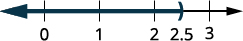用间隔符号书写。 $$(-\infty, 2.5)$$

 $$x \leq -\dfrac{3}{5}$$ 向左移动$$−\frac{3}{5}$$，然后在上面放一个括号$$−\frac{3}{5}$$。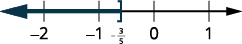用间隔符号书写。 $$\bigg( -\infty, \dfrac{3}{5}\bigg]$$

1. $$x>2$$
2. $$x\leq −1.5$$
3. $$x\geq \frac{3}{4}$$##### 示例$$\PageIndex{3}$$

1. $$x\leq −4$$
2. $$x\geq 0.5$$
3. $$x<−\frac{2}{3}$$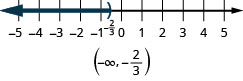图$$\PageIndex{3}$$

$$−3<x<4$$$$−6\leq x<−1$$$$0\leq x\leq 2.5$$

 $$-3 < x < 4$$ 介于$$−3$$和 4 之间的阴影。 在$$−3$$和 4 处加上圆括号。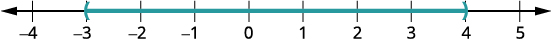用间隔符号书写。 $$(-3,4)$$

 $$-6 \leq x < -1$$ 介于$$−6$$和 −1 之间的阴影。 在 −1 处加上方括号$$−6$$ ，在 −1 处加上圆括号。用间隔符号书写。 $$[-6,1)$$

 $$0 \leq x \leq 2.5$$ 阴影介于 0 和 2.5 之间。 在 0 和 2.5 处放置一个括号。用间隔符号书写。 $$[0, 2.5]$$
##### 示例$$\PageIndex{5}$$

$$−2<x<1$$$$−5\leq x<−4$$$$1\leq x\leq 4.25$$##### 示例$$\PageIndex{6}$$

$$−6<x<2$$$$−3\leq x< −1$$$$2.5\leq x\leq 6$$## 求解线性不等式

##### 线性不等式

$\begin{array} {llll} {ax+b<c,} &{ax+b\leq c,} &{ax+b>c,} &{ax+b\geq c.} \\ \nonumber \end{array}$##### 不等式的加减特性

$\begin{array} {ll} {a+c<b+c} &{a−c<b−c} \\ {a+c>b+c} &{a−c>b−c} \\ \nonumber \end{array}$##### 不等式的乘法和除法特性

$\begin{array} {l} {\text{multiply or divide by a positive}} \\ \\ \space\space\space\space\text{if }a<b\text{ and }c>0\text{, then }ac<bc \text{ and }\frac{a}{c}<\frac{b}{c}. \\ \space\space\space\space\text{if }a>b\text{ and }c>0\text{, then }ac>bc \text{ and }\frac{a}{c}>\frac{b}{c}. \\ \\ \text{ multiply or divide by a negative } \\ \\ \space\space\space\space\text{if }a<b\text{ and }c<0\text{, then }ac>bc \text{ and }\frac{a}{c}>\frac{b}{c}.\\ \space\space\space\space\text{if }a>b\text{ and }c<0\text{, then }ac<bc \text{ and }\frac{a}{c}<\frac{b}{c}.\\ \nonumber \end{array}$

• 正数，不等式保持不变。
• 负数，不等式反转。

$x>a \text{ has the same meaning as } a<x \nonumber$

##### 示例$$\PageIndex{7}$$

$$x−\frac{3}{8}\leq \frac{3}{4}$$$$9y<54$$$$−15<\frac{3}{5}z$$将不等式的两边加上 3838。简化。在数字行上绘制解法。用间隔表示法写出解。将不等式的两边除以 9；由于 9 为正，因此不等式保持不变。简化。在数字行上绘制解法。用间隔表示法写出解。将不等式的两边乘以$$\frac{5}{3}$$。 因为$$\frac{5}{3}$$是正数，所以不等式保持不变。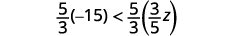简化。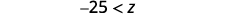用左边的变量重写。在数字行上绘制解法。用间隔表示法写出解。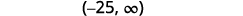$$p−\frac{3}{4}\geq \frac{1}{6}$$$$9c>72$$$$24\leq \frac{3}{8}m$$##### 示例$$\PageIndex{9}$$

$$r−\frac{1}{3}\leq \frac{7}{12}$$$$12d\leq 60$$$$−24<\frac{4}{3}n$$##### 示例$$\PageIndex{10}$$

$$−\frac{1}{3}m\geq \frac{6}{5}$$$$\frac{n}{−2} \geq 8$$将不平等的两面除以$$−\frac{1}{3}$$。 既然$$−\frac{1}{3}$$是负数，不等式就会逆转。简化。在数字行上绘制解法。用间隔表示法写出解。将不等式的两边乘以$$−2$$。 既然$$−2$$是负数，不等式就会逆转。简化。在数字行上绘制解法。用间隔表示法写出解。##### 示例$$\PageIndex{11}$$

$$−8q<32$$$$\frac{k}{−12} \leq 15$$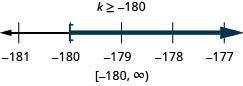##### 示例$$\PageIndex{12}$$

$$−7r\leq −70$$$$\frac{u}{−4}\geq −16$$从两边减去 11y11y 以收集左边 的变量。简化。将不等式的两边除以 −5、−5， 然后反转不等式。简化。在数字行上绘制解法。用间隔表示法写出解。##### 示例$$\PageIndex{15}$$##### 示例$$\PageIndex{16}$$

 $$8p+3(p−12)>7p−28$$ 尽可能简化每一面。 分发。 $$8p+3p−36>7p−28$$ 将相似的术语组合在一起。 $$11p−36>7p−28$$ $$7p$$从两边减去以收集左边的 变量，因为$$11>7$$。 $$11p−36−7p>7p−28−7p$$ 简化。 $$4p−36>−28$$ $$36$$向两边添加以收集右侧的 常量。 $$4p−36+36>−28+36$$ 简化。 $$4p>8$$ 将不等式的两边除以 $$4$$；不等式保持不变。 $$\dfrac{4p}{4}>\dfrac{8}{4}$$ 简化。 $$p>2$$ 在数字行上绘制解法。用间隔表示法写出解。 $$(2,\infty)$$
##### 示例$$\PageIndex{17}$$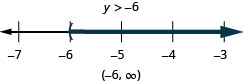##### 示例$$\PageIndex{18}$$##### 示例$$\PageIndex{19}$$

 尽可能简化每一面。 $$8x−2(5−x)<4(x+9)+6x$$ 分发。 $$8x−10+2x<4x+36+6x$$ 将相似的术语组合在一起。 $$10x−10<10x+36$$ $${\color{red}{10x}}$$从两边减去以收集左边的变量。 $$10x−10\,{\color{red}{-\,10x}}<10x+36\,{\color{red}{-\,10x}}$$ 简化。 $$−10<36$$ 这些都消失了，我们有了一个真实的 陈述。$$x$$ 不平等是一种身份。 解决方案都是实数。 在数字行上绘制解法。用间隔表示法写出解。 $$(−\infty,\infty)$$
##### 示例$$\PageIndex{20}$$##### 示例$$\PageIndex{21}$$##### 示例$$\PageIndex{22}$$

 $$\frac{1}{3}a−\frac{1}{8}a>\frac{5}{24}a+\frac{3}{4}$$ 将两边乘以液晶屏 24 以清除分数。 $${\color{red}{24}}\left(\dfrac{1}{3}a−\dfrac{1}{8}a\right)>\,{\color{red}{24}}\left(\dfrac{5}{24}a+\dfrac{3}{4}\right)$$ 简化。 $$8a - 3a > 5a + 18$$ 将相似的术语组合在一起。 $$5a > 5a + 18$$ $$5a$$从两边减去以收集左边的 变量。 $$5a \,{\color{red}{-\,5a}} > 5a \,{\color{red}{-\,5a}} + 18$$ 简化。 $$0 > 18$$ 这个说法是错误的。 不平等是矛盾的。 没有解决办法。 在数字行上绘制解法。用间隔表示法写出解。 没有解决办法。
##### 示例$$\PageIndex{23}$$##### 示例$$\PageIndex{24}$$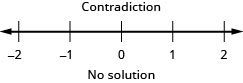## 转化为不等式并求解

$$>$$ $$\geq$$ $$<$$ $$\leq$$
\ (>\) “>\)” data-valign= “middle” class= “lt-math-17389” > 大

\ (\ geq\)” data-valign= “middle” >大于或等

\ 小于<\)” data-valign="middle"> 小于

\ (\ leq\)” data-valign= “middle” >小于或等

##### 示例$$\PageIndex{25}$$

$\text{Twenty-seven less than }x\text{ is at least }48.\nonumber$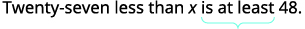翻译。 $$x - 27 \geq 48$$ 求解-向两边加 27。 $$x - 27 \, {\color{red}{+\, 27}} \geq 48 \, {\color{red}{+\, 27}}$$ 简化。 $$x \geq 75$$ 数字线上的图形。用间隔符号书写。 $$[75, \infty)$$

$\text{Nineteen less than } p \text{ is no less than }47.\nonumber$##### 示例$$\PageIndex{27}$$

$\text{Four more than }a\text{ is at most }15.\nonumber$## 求解具有线性不等式的应用程序

##### 示例$$\PageIndex{28}$$

$$\begin{array} {ll} {\textbf{Step 1. Read }\text{ the problem.}} &{} \\ {\textbf{Step 2. Identify }\text{what you are looking for.}} &{\text{the maximum number of tablets Dawn can buy}} \\ {\textbf{Step 3. Name}\text{ what you are looking for.}} &{} \\ {} &{} \\ {} &{\text{Let }n= \text{ the number of tablets.}} \\ {\text{Choose a variable to represent that}} &{} \\{\text{quantity.}} &{} \\ {\textbf{Step 4. Translate.}\text{Write a sentence that gives the}} &{} \\ {\text{information to find it.}} &{254.12\text{ times the number of tablets is}} \\ {} &{\text{no more than }4,000.} \\ {} &{\space\space\space\space\space\space\space\space\space\space\space\space 254.12n\leq 4000} \\ {\text{Translate into an inequality.}} &{} \\ {\textbf{Step 5. Solve }\text{the inequality.}} &{} \\ {} &{\space\space\space\space\space\space\space\space\space\space\space\space\space\space\space\space\space\space\space\space n\leq 15.74} \\ {} &{\space\space\space\space\space\space\space\space\space\space\space\space\space\space\space\space\space\space\space\space n\leq 15} \\{\text{But }n\text{ must be a whole number of}} &{} \\ {\text{tablets, so round to }15.} &{} \\ {\textbf{Step 6. Check }\text{the answer in the problem}} &{} \\ {\text{and make sure it makes sense.}} &{} \\ {} &{} \\ {} &{} \\ {\space\space\space \text{Rounding down the price to } 250, 15} &{} \\ {\space\space\space \text{tablets would cost }3,750,\text{ while } 16} &{} \\ {\space\space\space \text{tablets would be }4,000.\text{So a}} &{} \\ {\space\space\space \text{maximum of 15 tablets at }254.12} &{} \\ {\space\space\space \text{seems reasonable.}} &{} \\ {\textbf{Step 7. Answer }\text{the question with a complete sentence.}} &{\text{Dawn can buy a maximum of 15 tablets.}} \\ \end{array}$$

##### 示例$$\PageIndex{31}$$

Taleisha的电话套餐每月花费28.80美元，外加每条短信0.20美元。 她可以发送/接收多少条短信，并且每月的电话账单不超过50美元？

$$\begin{array} {ll} {\textbf{Step 1. Read }\text{the problem.}} &{} \\ {\textbf{Step 2. Identify }\text{what you are looking for.}} &{\text{the number of text messages Taleisha can make}} \\ {\textbf{Step 3. Name }\text{what you are looking for.}} &{} \\ {} &{} \\ {} &{\text{Let }t= \text{the number of text messages.}} \\ {\text{Choose a variable to represent that}} &{} \\ {\text{quantity.}} &{} \\ {\textbf{Step 4. Translate }\text{Write a sentence that}} &{} \\ {\text{gives the information to find it.}} &{28.80\text{ plus }0.20\text{ times the number of}} \\ {} &{\text{text messages is less than or equal to }50.} \\ {} &{28.80+0.20t \leq 50} \\ {\space\space\space \text{Translate into an inequality.}} &{} \\ {\textbf{Step 5. Solve }\text{the inequality.}} &{} \\ {} &{\space\space\space\space\space\space\space\space\space\space\space\space\space\space\space 0.2t\leq 21.2} \\ {} &{\space\space\space\space\space\space\space\space\space\space\space\space\space\space\space\space\space\space\space\space t\leq 106\text{ text messages}} \\ {} &{} \\ {\textbf{Step 6. Check }\text{the answer in the problem}} &{} \\ {\text{and make sure it makes sense.}} &{} \\ {} &{} \\ {} &{} \\{\space\space\space \text{Yes, }28.80+0.20(106)=50.} &{} \\ {\textbf{Step 7. Write }\text{a sentence that answers the question.}} &{} \\ {} &{\text{Taleisha can send/receive no more than}} \\ {} &{106\text{ text messages to keep her bill no}} \\ {} &{\text{more than } 50.} \\ \end{array}$$

##### 示例$$\PageIndex{33}$$

Rameen的取暖费为每月5.42美元，加上每热1.08美元。 如果 Rameen 想让取暖费最高为 87.50 美元，他能使用多少保温器。

Rameen的取暖费为每月5.42美元，加上每热1.08美元。 如果 Rameen 想让取暖费最高为 87.50 美元，他能使用多少保温器。

##### 示例$$\PageIndex{34}$$

Felicity 有一家书法生意。 她每张结婚请柬收取2.50美元的费用。 她每月的开支是650美元。 她必须写多少邀请函才能赚取每月至少2,800美元的利润？

$$\begin{array} {ll} {\textbf{Step 1. Read }\text{the problem.}} &{} \\ {\textbf{Step 2. Identify }\text{what you are looking for.}} &{\text{the number of invitations Felicity needs to write}} \\ {\textbf{Step 3. Name }\text{what you are looking for.}} &{\text{Let }j=\text{ the number of invitations.}} \\ {} &{} \\ {\space\space\space\text{Choose a variable to represent it.}} &{} \\ {\textbf{Step 4. Translate. }\text{Write a sentence that}} &{} \\ {\text{gives the information to find it.}} &{2.50 \text{ times the number of invitations}} \\ {} &{\text{minus }650\text{ is at least }2,800.} \\ {} &{\space\space\space 2.50j−650\geq 2,800} \\ {\space\space\space \text{Translate into an inequality.}} &{} \\ {\textbf{Step 5. Solve }\text{the inequality.}} &{} \\ {} &{\space\space\space\space\space\space\space\space\space\space\space\space\space\space\space 2.5j\geq 3,450} \\ {} &{\space\space\space\space\space\space\space\space\space\space\space\space\space\space\space\space\space\space\space\space j\geq 1,380 \text{ invitations}} \\ {\textbf{Step 6. Check }\text{the answer in the problem}} &{} \\ {\text{and make sure it makes sense.}} &{} \\ {} &{} \\ {} &{} \\ {\space\space\space \text{If Felicity wrote }1400\text{ invitations, her}} &{} \\ {\space\space\space \text{profit would be }2.50(1400)−650, \text{or}} &{} \\ {\space\space\space 2,850.\text{ This is more than }2800.} &{} \\ {\textbf{Step 7. Write }\text{a sentence that answers the question.}} &{\text{Felicity must write at least }1,380\text{ invitations.}} \\ \end{array}$$

##### 示例$$\PageIndex{35}$$

Caleb 有一家宠物看护公司。 他每小时收费32美元。 他每月的开支为2,272美元。 他必须工作多少小时才能赚取每月至少800美元的利润？

Caleb 必须工作至少 96 个小时。

##### 示例$$\PageIndex{37}$$

$$\begin{array} {ll} {} &{} \\ {\textbf{Step 1. Read }\text{the problem.}} &{} \\ {\textbf{Step 2. Identify }\text{what you are looking for.}} &{\text{the number of hours Malik must tutor}} \\ {\textbf{Step 3. Name }\text{what you are looking for.}} &{} \\ {} &{} \\ {} &{\text{Let }h=\text{ the number of hours.}} \\ {\space\space\space\space\space\space\space \text{Choose a variable to represent that}} &{} \\ {\space\space\space\space\space\space\space \text{quantity.}} &{} \\ {\textbf{Step 4. Translate. }\text{Write a sentence that}} &{} \\ {\text{gives the information to find it.}} &{} \\ {} &{\text{The expenses must be less than or equal to}} \\{} &{\text{the income. The cost of airfare plus the}} \\{} &{\text{cost of food and sightseeing and the hotel}} \\{} &{\text{bill must be less than the savings plus the}} \\{} &{\text{amount earned tutoring.}} \\{} &{} \\ {} &{} \\ {} &{} \\ {} &{} \\ {\space\space\space\space\space\space\space \text{Translate into an inequality.}} &{525+780+95(6)\leq 840+45h} \\ {\textbf{Step 5. Solve }\text{the inequality.}} &{} \\ {} &{} \\ {} &{\space\space\space\space\space\space\space\space\space\space\space\space\space\space\space\space 1,875\leq 840+45h} \\ {} &{\space\space\space\space\space\space\space\space\space\space\space\space\space\space\space\space 1,035\leq 45h} \\ {} &{\space\space\space\space\space\space\space\space\space\space\space\space\space\space\space\space\space\space\space\space 23\leq h} \\ {} &{\space\space\space\space\space\space\space\space\space\space\space\space\space\space\space\space\space\space\space\space\space\space\space h\geq 23} \\ {} &{} \\ {} &{} \\ {\textbf{Step 6. Check }\text{the answer in the problem}} &{} \\ {\text{and make sure it makes sense.}} &{} \\ {\text{We substitute 23 into the inequality.}} &{} \\ {} &{} \\ {} &{} \\ {\space\space\space\space\space\space\space\space 1,875\leq 840+45h} &{} \\ {\space\space\space\space\space\space\space\space 1,875\leq 840+45(23)} &{} \\ {\space\space\space\space\space\space\space\space 1,875\leq 1875} &{} \\ {\textbf{Step 7. Write }\text{a sentence that answers the question.}} &{\text{Malik must tutor at least }23\text{ hours.}} \\ \end{array}$$

##### 示例$$\PageIndex{39}$$

Josue想在明年春天和朋友一起去10晚的公路旅行。 他将花费180美元购买汽油，450美元购买食物，每晚花费49美元。 他有520美元的储蓄，每条车道铲雪可以赚30美元。 他必须铲多少车道才能有足够的钱支付旅行费用？

Josue 必须铲掉至少 20 条车道。

## 关键概念

• 不等式、数字线和间隔记法
$$x>a \quad x\geq a\quad x<a\quad x\leq a$$• 线性不等式
• 线性不等式是一个变量中的不等式，可以用以下形式写成，其中 abc 是实数，并且$$a\neq 0$$

$ax+b<c, \qquad ax+b\leq c, \qquad ax+b>c, \qquad ax+b\geq c.\nonumber$

• 不等式的加减特性
• 对于任何数字 abc，如果 a<b，那么 na<b，那么

$\begin{array} {ll} {a+c<b+c} &{a−c<b−c} \\ {a+c>b+c} &{a−c>b−c} \\ \end{array} \nonumber$

• 我们可以从不等式的两边加上或减去相同的数量，但仍然保持不等式。
• 不等式的乘法和除法特性
• 对于任何数字 abc
$$\begin{array} {l} \text{multiply or divide by a }\textbf{positive} \\ \\ \space\space\space\space\space\space\space\text{if }a<b\text{ and }c>0,\text{ then } ac<bc\text{ and }\frac{a}{c}<\frac{b}{c}. \\ \space\space\space\space\space\space\space\text{if }a>b\text{ and }c>0,\text{ then } ac>bc\text{ and }\frac{a}{c}>\frac{b}{c}. \\ \text{multiply or divide by a }\textbf{negative} \\ \\ \space\space\space\space\space\space\space\text{if }a<b\text{ and }c<0,\text{ then } ac>bc\text{ and }\frac{a}{c}>\frac{b}{c}. \\ \space\space\space\space\space\space\space\text{if }a>b\text{ and }c<0,\text{ then } ac<bc\text{ and }\frac{a}{c}<\frac{b}{c}. \\ \end{array}$$
• 表示不平等的短语
$$>$$ $$\geq$$ $$<$$ $$\leq$$
\ (>\) “>大于大

于大

于大于

超过
\ (\ geq\)” data-valign= “middle” >大于或等

于至少不小

于最小值

\ 小于<\)” data-valign="middle"> 小于

小于小于

小于

小于
\ (\ leq\)” data-valign= “middle” >小于或等

于最多不大

于最大值